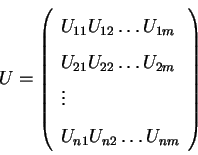Next: Temporal Utilities Up: Decision Making Previous: Outcomes

# Utility Function

A utility function transforms an outcome into a numerical value and measures the worth of an outcome. The utility of an outcome may be negative or positive. This utility function may be a simple table, a linear function, or a more complex function. The outcome matrix is converted to the utility matrix using the utility function.

Let U be the utility matrixwhere Uij is the utility of the ith alternative task dealing with the jth objective.

Ronald LeRoi Burback
1998-12-14